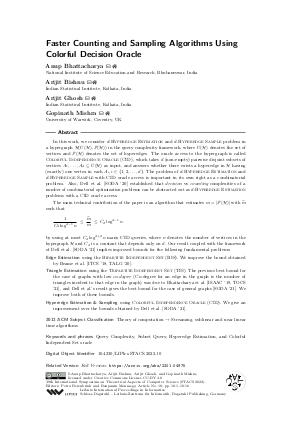Document# Faster Counting and Sampling Algorithms Using Colorful Decision Oracle

### Authors Anup Bhattacharya, Arijit Bishnu, Arijit Ghosh, Gopinath Mishra## File

LIPIcs.STACS.2022.10.pdf
• Filesize: 0.79 MB
• 16 pages

## Cite As

Anup Bhattacharya, Arijit Bishnu, Arijit Ghosh, and Gopinath Mishra. Faster Counting and Sampling Algorithms Using Colorful Decision Oracle. In 39th International Symposium on Theoretical Aspects of Computer Science (STACS 2022). Leibniz International Proceedings in Informatics (LIPIcs), Volume 219, pp. 10:1-10:16, Schloss Dagstuhl - Leibniz-Zentrum für Informatik (2022)
https://doi.org/10.4230/LIPIcs.STACS.2022.10

## Abstract

In this work, we consider d-Hyperedge Estimation and d-Hyperedge Sample problem in a hypergraph H(U(H),F(H)) in the query complexity framework, where U(H) denotes the set of vertices and F(H) denotes the set of hyperedges. The oracle access to the hypergraph is called Colorful Independence Oracle (CID), which takes d (non-empty) pairwise disjoint subsets of vertices A₁,…, A_d ⊆ U(ℋ) as input, and answers whether there exists a hyperedge in H having (exactly) one vertex in each A_i, i ∈ {1,2,…,d}. The problem of d-Hyperedge Estimation and d-Hyperedge Sample with CID oracle access is important in its own right as a combinatorial problem. Also, Dell et al. [SODA '20] established that decision vs counting complexities of a number of combinatorial optimization problems can be abstracted out as d-Hyperedge Estimation problems with a CID oracle access. The main technical contribution of the paper is an algorithm that estimates m = |F(H)| with m̂ such that 1/(C_{d)log^{d-1} n) ≤ m̂/m ≤ C_{d} log ^{d-1} n. by using at most C_{d}log ^{d+2} n many CID queries, where n denotes the number of vertices in the hypergraph H and C_d is a constant that depends only on d}. Our result coupled with the framework of Dell et al. [SODA '21] implies improved bounds for the following fundamental problems: Edge Estimation using the Bipartite Independent Set (BIS). We improve the bound obtained by Beame et al. [ITCS '18, TALG '20]. Triangle Estimation using the Tripartite Independent Set (TIS). The previous best bound for the case of graphs with low co-degree (Co-degree for an edge in the graph is the number of triangles incident to that edge in the graph) was due to Bhattacharya et al. [ISAAC '19, TOCS '21], and Dell {et al.}’s result gives the best bound for the case of general graphs [SODA '21]. We improve both of these bounds. Hyperedge Estimation & Sampling using Colorful Independence Oracle (CID). We give an improvement over the bounds obtained by Dell et al. [SODA '21].

## Subject Classification

##### ACM Subject Classification
• Theory of computation → Streaming, sublinear and near linear time algorithms
##### Keywords
• Query Complexity
• Subset Query
• Hyperedge Estimation
• and Colorful Independent Set oracle

## Metrics

• Access Statistics
• Total Accesses (updated on a weekly basis)
0

## References

1. Paul Beame, Sariel Har-Peled, Sivaramakrishnan Natarajan Ramamoorthy, Cyrus Rashtchian, and Makrand Sinha. Edge Estimation with Independent Set Oracles. In Proceedings of the 9th Innovations in Theoretical Computer Science Conference, ITCS, volume 94, pages 38:1-38:21, 2018.2. Paul Beame, Sariel Har-Peled, Sivaramakrishnan Natarajan Ramamoorthy, Cyrus Rashtchian, and Makrand Sinha. Edge Estimation with Independent Set Oracles. ACM Trans. Algorithms, 16(4):52:1-52:27, 2020.3. Anup Bhattacharya, Arijit Bishnu, Arijit Ghosh, and Gopinath Mishra. Triangle Estimation Using Tripartite Independent Set Queries. In Proceedings of the 30th International Symposium on Algorithms and Computation, ISAAC, volume 149, pages 19:1-19:17, 2019.4. Anup Bhattacharya, Arijit Bishnu, Arijit Ghosh, and Gopinath Mishra. On Triangle Estimation Using Tripartite Independent Set Queries. Theory Comput. Syst., 65(8):1165-1192, 2021.5. Anup Bhattacharya, Arijit Bishnu, Arijit Ghosh, and Gopinath Mishra. Faster Algorithms for Estimating and Sampling using Colorful Decision Oracle, 2022. URL: http://arxiv.org/abs/2201.04975.
6. Arijit Bishnu, Arijit Ghosh, Sudeshna Kolay, Gopinath Mishra, and Saket Saurabh. Parameterized Query Complexity of Hitting Set Using Stability of Sunflowers. In Proceedings of the 29th International Symposium on Algorithms and Computation, ISAAC, volume 123, pages 25:1-25:12, 2018.7. Holger Dell and John Lapinskas. Fine-Grained Reductions from Approximate Counting to Decision. In Proceedings of the 50th Annual ACM SIGACT Symposium on Theory of Computing, STOC, pages 281-288, 2018.8. Holger Dell and John Lapinskas. Fine-Grained Reductions from Approximate Counting to Decision. ACM Trans. Comput. Theory, 13(2):8:1-8:24, 2021.9. Holger Dell, John Lapinskas, and Kitty Meeks. Approximately counting and sampling small witnesses using a colourful decision oracle. In Proceedings of the 2020 ACM-SIAM Symposium on Discrete Algorithms, SODA, pages 2201-2211, 2020.10. Devdatt P. Dubhashi and Alessandro Panconesi. Concentration of Measure for the Analysis of Randomized Algorithms. Cambridge University Press, 2009.11. Talya Eden, Amit Levi, Dana Ron, and C. Seshadhri. Approximately counting triangles in sublinear time. SIAM J. Comput., 46(5):1603-1646, 2017. URL: https://doi.org/10.1137/15M1054389.
12. Talya Eden, Dana Ron, and C. Seshadhri. On Approximating the Number of k-Cliques in Sublinear Time. SIAM J. Comput., 49(4):747-771, 2020.13. Uriel Feige. On Sums of Independent Random Variables with Unbounded Variance and Estimating the Average Degree in a Graph. SIAM J. Comput., 35(4):964-984, 2006.14. Oded Goldreich and Dana Ron. Approximating Average Parameters of Graphs. Random Struct. Algorithms, 32(4):473-493, 2008.X

Feedback for Dagstuhl Publishing